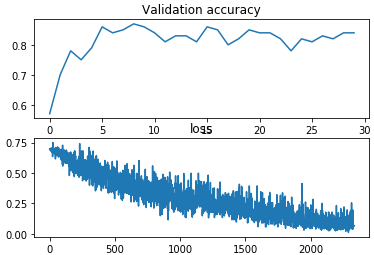# Cats Vs Dogs CNN has pretty low accuracy

``````import time as t
import torch
import torch.nn as nn
import torch.nn.functional as F
import torch.optim as optim
from torchvision import datasets, transforms
from random import randint
import numpy as np
import tqdm
from matplotlib import pyplot as plt

#%%
#
#(tensor(1x50x50 image),target),....
data = []
for f in from_file:
entry = []
img = f/255
tar = f
img_t = torch.Tensor([img])
entry.append(img_t)
entry.append(tar)
data.append(entry)
#%%
trainset = torch.utils.data.DataLoader(data[100:], batch_size = 64,
shuffle = True)
testset = torch.utils.data.DataLoader(data[:100], batch_size = 1,
shuffle = True)
#%%

class Net(nn.Module):

def __init__(self):
super(Net, self).__init__()
self.conv1 = nn.Conv2d(1, 50, kernel_size=3)
self.conv2 = nn.Conv2d(50, 50, kernel_size=3)
self.conv3 = nn.Conv2d(50, 50, kernel_size=3)
self.conv4 = nn.Conv2d(50, 55, kernel_size=3)
self.conv5 = nn.Conv2d(55, 55, kernel_size=3)
self.mp = nn.MaxPool2d(2)
self.fc = nn.Linear(3520, 2)

def forward(self, x):
in_size = x.size(0)
x = F.relu(self.mp(self.conv1(x)))
x = F.relu(self.conv2(x))
x = F.relu(self.conv3(x))
x = F.relu(self.conv4(x))
x = F.relu(self.mp(self.conv5(x)))
x = x.view(in_size, -1)  # flatten the tensor
x = self.fc(x)
return x

loss_func = nn.CrossEntropyLoss()
net = Net().cuda()

print('net created')

#%%
def test():
#testing time
net.eval()
total = 0
correct = 0

#print('validation ongoing')

for dat in testset:
x,y = dat
x = x.cuda()
output = net(x)
y = list(y)
values, indices = output.max(0)
y = int(y)
indices = int(indices)
#   print('True Value = ',y)
#   print('Prediction = ',indices)
#   print('\n______________________________________')
if y == indices:
correct += 1

total += 1
#print((correct/total)*100,'validation accuracy')
return (correct/total)
#%%
count = 0
acc = []
losses = []
for epoch in tqdm.tqdm(range(30)):
net.train()#training mode
for data in trainset:
t1 = t.time()
x,y = data
x = x.cuda()
y = y.cuda()
output = net(x)
#print(output,y)
loss = loss_func(output, y)
#print(output,y)
loss.backward()
optimizer.step()

count  += 1
if count%5 == 0:
#print(round(float(loss),4),'|||',round((t.time() - t1)*1000,3),'ms/batch    ',epoch)
count  = 0
losses.append(float(loss))
acc.append(test())
#%%
fig = plt.figure()
plt.subplot(2,1,1)
plt.plot(acc)
plt.title('Validation accuracy')
plt.subplot(2,1,2)
plt.plot(losses)
plt.title('loss')
print(max(acc))
plt.show()
``````

With this CNN architecture I only ever get close to 87% validation accuracy. Increasing the number of conv layers actually made the accuracy worse.

This is a plot I’m generatingAfter every epoch, im putting the net into eval mode and trying out the validation data to get accuracy. In this case i get 87%.

Any advice on how to refine and improve my net would be greatly appreciated.
Thanks!

Hello Maths!

Cats Vs Dogs also has a pretty low rating (rotten) on Rotten Tomatoes.

Best.

K. Frank

It’s not a bad accuracy at all, but you could try increasing the number of kernels (out_channels) in conv layers in a standard way like [1,32,64,128,256,512] . Also you could try training a ResNet18 and compare the results.

``````from torchvision import models
net = models.resnet18(pretrained=False)
``````